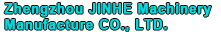Technical consultation：

sales@drymixer.com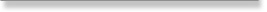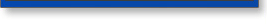Mixing Model and JINHE Indexmoxing

# Mixing Models and JINHE Mixing Indexinput time：2017-06-28     hits：19

Flowing powder liquid mixing model

First, the origin of the hybrid model

In the past, the study of powder mixing was carried out in a static process. However, due to the different size, variety and variety of powders, the mixing process was carried out under the condition of continuous displacement of powder. Powder mixing is a complicated and difficult job.
After many years of research, we have observed that under the intense external force, the displaced and turbulent powders show very similar movement characteristics to the liquid.
Therefore, the complicated and difficult research work can be made clear and easy by establishing the powder-liquid mixing model to explain and analyze the powder mixing motion.

Second, flowing powder liquid mixing model

1, the definition
Definition: Under the action of external force, the powder in the container will be intensely moved, and the powders will mix with each other. During the mixing process, the liquefaction characteristics appear. That is, the powder presents "floating", "sinking" "Floating" and other phenomena, the law of movement of powder and liquid movement roughly the same. Different characteristics of the powder in the "liquid" showed the corresponding movement trend.
Explanation: 1) Liquid features such as upward floating, suspended in the middle, sinking at the bottom of the phenomenon also appear in sports powder. Use these phenomena to explain the powder mix more intuitive, accurate and easy to understand. When we take a glass bottle containing half the powder for intense shaking, we can see that the powder flows like a liquid, floating and shocking.
2) The liquid "liquid" density in the model is the mean of the density of all the powders participating in the mixing. The closer a powder density is to the average, the easier it is for the powder to mix into the bulk powder.
2, the movement of the powder in the mixed model features (below)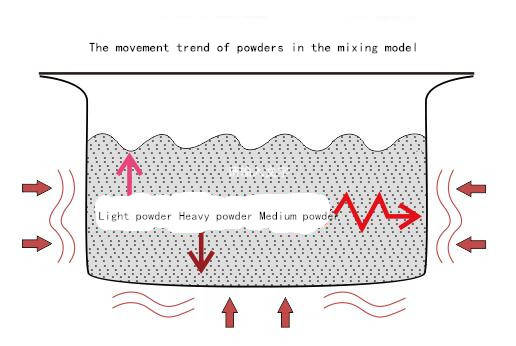1) The powder flows and diffuses from high to low under the action of gravity;
2) light powder, fine powder floating up, heavy powder, large particles of powder sink to the bottom;
3) the more intense powder movement liquid phenomenon is more obvious;
4) The better the fluidity of the liquid, the more obvious the liquefaction phenomenon.
Third, the significance of sports powder liquid model

The kinetic powder liquefaction mixing model gives the theoretical basis for analyzing and studying the powder mixing technology. On this basis we can get the following uses:
1) Accurately find out the reason and influence of the powder characteristics in powder mixing
2) is to guide the mixing experiment, the basis for the development of mixed technology
3) Laying a rare theoretical foundation for quantifying and deepening the research on powder mixing technology
4) It leads to an important composite index, which has practical significance for accurately guiding powder mixing

Fourth, through the mixed model analysis of the physical characteristics of the mixed powder

It is intuitive and convenient to analyze the influence of the physical properties of the powder on the mixing based on the liquidized mixing model of the moving powder.
1, the impact of powder density on mixing
Powder density is one of the important powder characteristics that affects the mixing process. According to the theory of liquefaction, heavier powders easily sink, lighter powders tend to float, and only moderately weighted powders are better suspended in the main powder for blending and penetration with each other to achieve uniform mixing purpose.
Among them, the proper proportion of powder means that the density mean of all the powders mixed with each other is similar. And the average value is too light or too heavy powder will give mixed difficult, too light, too heavy means that the average density of the powder with the whole difference of more than several times.
Usually the density value below 0.1 is easy to cause obvious difficulties mixing work. Therefore, in order to achieve the purpose of mixing evenly, the difference in density between different powders needs to be controlled too much.
2, the impact of particle size on the mixing
The average particle size of the powder is also an important characteristic that affects mixing:
1) The smaller the particle size, the finer the powder, the easier it is to float upward when mixing, whereas the larger the particle size, the more coarse the powder, the easier it is to sink to the bottom during mixing.
2) because it is the average particle size, and actually participate in the mixed powder particle size is different, if the ratio of the size of a certain powder size reaches several times, there will be small particles of the powder on the floating , The phenomenon of large size powder sinking.
Usually less than 50 mesh size and more than 800 mesh will cause obvious difficulties mixing work. Therefore, in order to achieve the purpose of mixing evenly, the difference in particle size between powders needs to be controlled.
3, the impact of powder mobility on the mix
In principle, the better powder fluidity, the higher the efficiency of powder mixing, but in the actual operation should pay attention to the following points:
1) the fluidity of the powder on the mixing effect:
Positive effect: high mixing efficiency. According to the theory of liquefaction mixing model, the faster the powder flow rate is, the more the powders are mixed with each other and the higher the permeation efficiency is, and therefore the easier the mixing is achieved.
Negative impact: According to the theory of liquefaction mixing model, powder in the mixing process due to the powder flow is better, will strengthen due to differences in the characteristics of the powder produced a new floating or sinking phenomenon; and after mixing, the powder in the Loading and unloading, transportation vibration prone to secondary segregation, stratification, resulting in new uneven.
2) poor mobility of the powder on the impact of mixing:
Positive impact: strong holding power of powder, once all kinds of powder diffusion uniformity (that is, mixed), it is not easy to produce new segregation, delamination and new uneven phenomenon.
Negative impact: According to the theory of liquefaction mixing model, powder in the mixing process due to poor powder flow, powder flow slow mixing will make it difficult to reduce the mixing efficiency, not easy to achieve the purpose of mixing.
3) In practical work, the liquidity is too good or too poor. The mixing of powders is actually a mixing process. (The relationship between mixing and flowability is shown in the figure below.) A powerful mixing equipment is needed to solve the problem.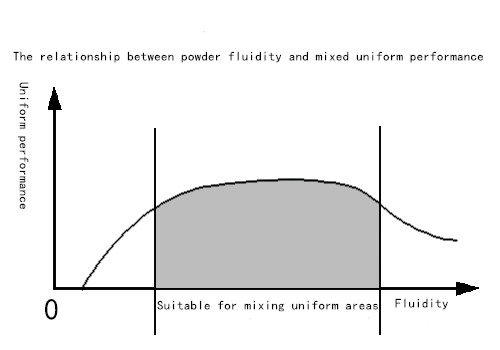4) The fluidity of powder is determined by the fluidity of the main powder with a large content of ingredients.
4, the product of powder density and particle size - the significance of the equilibrium value
The two eigenvalues ??are analyzed separately for their influence, but in fact the product of two eigenvalues ??- the equilibrium value to analyze the mixing of the powder
The effect of the effect is more accurate and direct.
1) Feature Product: Feature Product (Equilibrium Value) = Density * Particle Size
2) For example:
Powder a - density 1.0, particle size 28 microns
Characteristic product = 1.0 * 28 = 28
Powder b - density 0.2, particle size 150 microns
Characteristic product = 0.2 * 150 = 30
① From the density analysis of a powder weight has the tendency to sink, b pink light upward trend, a difference of 5 times the density, mixing difficulties.
②. From the particle size analysis of a fine powder floating upward trend, b coarse powder with a downward trend, a difference of 5.3 times the size, mixing difficulties.
③. And from the product of the characteristic analysis of a powder, b powder close, indicating that the mixing can be carried out smoothly. This is because the proportion of a powder sinking trend and the trend of particle size offset offset, compared with the b composite features are relatively balanced.
3) Application: It can be seen from the above example that although a powder is heavy and easy to sink but its particle size is very small, its floating performance can make up for the downward trend, and a and b are close to each other. Therefore, Value) analysis to determine the hybrid movement is a more realistic approach.

Mixed gold composite index

First, the definition of hybrid gold composite index

1 Overview
Metallurgical composite index is based on the movement of powder liquid mixture model based on research and development.
Sports powder liquid mixture model is to describe the various hybrids in the dynamic mixing of their movement trend. The tendency of "floating" and "sinking" segregation movement is more difficult to mix homogeneously.
The composite index is the mathematical method to quantify the strength of the trend of this trend has become the basis for the guidance of mixed work. So mastering the calculation and use of composite index, you can easily control the smooth mixing of powder.
2. Powder property value setting
Let each powder can be its proportion multiplied by the average particle size as its characteristic value.
Expressed as: B × D
Of which: B = the proportion of powder
D = average particle size of the powder
3. To be mixed powder characteristics of the average setting
Let the average value of the characteristics of the batch mixed powder is H, and Ki is the proportion (percentage) of the ith kind of powder in the batch mixed powder, and the larger the proportion is, The greater the average effect of the characteristics of the powder to be mixed.
According to the above guidelines, the average of the characteristics can be expressed by the following equation:
H = B1 × D1 × K1 + ... + Bi × Di × Ki + ... + Bn × Dn × Kn
Summarized as: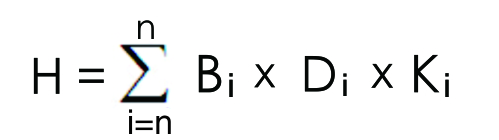Of which: Bi = the proportion of the i-th powder;
Di = particle size of the ith powder;
Ki = the percentage of i-th powder.
i = 1 ~ n
That is to say that the average value of the properties of the powders to be mixed is equal to the product of the specific gravity of each powder multiplied by the average particle diameter of the powder and multiplied by the product of its proportion in the powder mixture Sum together.
4 gold composite index set
Definition: The specified composite powder metallurgical index is the characteristic value of the powder to be mixed with the powder characteristics of the average ratio.
Therefore, the following formula shows:
\
Where: H = B1 × D1 × K1 + ... + Bi × Di × Ki + ... + Bn × Dn × Kn
In the formula: B1, D1 and K1 represent the specific gravity, the average particle diameter and the percentage of the first powder;
Bi, Di and Ki represent the specific gravity, the average particle diameter and the percentage of the ith kind of powder;
n said the number of mixed powder;
H represents the average value of the batch mixed powder characteristics;
Ji said the i-th powder mixed composite index;
When i = 1 ~ n can be calculated for each of the powder composite index J1 ~ Jn.
5. Example Calculate the composite index
For example, there are three kinds of powders to be mixed, and the first step is to measure the quantifiable physical indexes of each kind of powder: the specific gravity, the average particle size and the proportions in the batch of mixed powders are as follows:
The first powder B1 = 1.1 D1 = 0.01 mm K1 = 30%
The second powder B2 = 0.9 D2 = 0.015 mm K2 = 60%
The third powder B3 = 2.1 D3 = 0.03mm K3 = 10%
The second step to calculate the average value of properties:
H = 1.1 × 0.01 × 30% + 0.9 × 0.015 × 60% + 2.1 × 0.03 × 10%
= 0.0033 + 0.0081 + 0.0063
= 0.0177
The third step and then calculate the three kinds of powder metallurgical hybrid index:
J1 = 1.1 × 0.01 ÷ 0.0177≈0.62
J2 = 0.9 × 0.015 ÷ 0.0177≈0.76
J3 = 2.1 × 0.03 ÷ 0.0177≈3.56

Second, interpretation and application

1. Mixed interpretation of the metal
1) Each of the specified powders to be mixed has a corresponding composite index
After the powder to be mixed is determined, the corresponding composite index of each powder can be calculated by the above formula. The larger the composite index, the larger the particle size or the larger the specific gravity of the powder. The smaller the gold composite index is, the smaller the particle size or the lighter the specific gravity.
2) The value of the gold compounding index of a powder is related to its own properties and to other properties of the powder to be mixed together
If you change the index of a powder will affect the rest of each of the powder metallurgical composite index, reduce or increase the powder will change the rest of each powder metallurgical mixed index.
2 gold composite index of practical significance
1) The meaning of the value of the gold composite index value
① The value of the composite index is equal to 1, indicating that the specific gravity and the average particle size of the powder are basically the same as the average value of the powder to be mixed, indicating that the powder is easy to participate in the mixing.
② The value of the composite index is less than 1, indicating that the powder is lighter or thinner than the average value of the powder to be mixed, and if the value is below 0.3, it is difficult to mix uniformly.
③ The value of the composite index is greater than 1, indicating that the powder and the average powder to be mixed compared to the average, relatively heavy or relatively large particle size, the value of 3.0 or more, the participation of mixed more difficult to mix evenly. Usually we can use the method of refining the average particle size of the powder to reduce the value of its composite index, so that the mixing becomes easy.
2) quantitative analysis of the complexity of the mixed process by the ratio of the size of the gold composite index
In the various powders to be mixed using the above formula to calculate the gold content of each mixed powder composite index.
① select the largest of which the composite gold combination index and the minimum composite index, and calculate the ratio of Z:
\
Where JMAX is the largest batch mixed powder composite index value, JMIN is the smallest gold composite blend index value.
②. Differentiation ratio z to determine the ease of mixing process:
Through a large number of experiments, the following conclusions are drawn: the ratio z is greater than 3, which indicates that the difference between the two powders is large and the difficulty of mixing begins to increase. If the ratio z is greater than 6, the difference between the two powders is particularly large Even mixing is a hard job to achieve.
3) meaning of the composite index
The difference between the composite index is the key to the index application. The smaller the difference is, the closer the physical indices between the powders are, and the less the heterogeneity is, the more homogeneous the powder is. On the contrary, the greater the difference between the gold composite index indicates that there is a difference between the powder mixing, powder appeared between the light and heavy powder mixing process problems, mixing difficulties. Therefore, we can use the gold composite index to guide the powder mixing process smoothly.
The composite index is a theory put forward by our company's designer team through many years of powder mixing experience, scientific summary and independent research.
The introduction of the gold composite index is to quantitatively describe the degree of difficulty of the hybrid process, which provides a very effective and easy-to-grasp scientific guidance method for the powder mixing work, and fills the theory of the powder mixing process at home and abroad A blank.
3. The application of composite index
Co-ming composite index to guide the mixing work has very good practical significance.
When the variational ratio of the gold mixed index z is within 3, the mixing task is easy to complete and the mixing can be carried out using conventional mixing equipment.
When the ratio of composite gold composite index z is greater than 3 or even more than 10, then the mixing difficulty gradually enhanced or even difficult to achieve the purpose of mixing. For this situation can be used in the following ways:
1) The larger, heavier particles in the powder are ground to reduce the index difference ratio z to achieve an easy mixture.
2) The use of forced mixing function to solve the problem of the mixer, such as dual-motion mixer. The double barrel mixer barrel tumble and coercion ability can inhibit the powder "floating" and "sinking" trend, so as to achieve the purpose of forced mixing evenly.
4. Application examples
1) Calculated before processing:
Also with the aforementioned three kinds of powder as an example, and has calculated the corresponding composite index:
The first powder B1 = 1.1 D1 = 0.01 mm J1 ≈ 0.62
The second powder B2 = 0.9 D2 = 0.015 mm J2 ≈ 0.76
The third powder B3 = 2.1 D3 = 0.03mm J3≈3.56
Characteristic average: H = 0.0177
Select the maximum and minimum composite gold composite index, calculate their size ratio of the gold mixed index:
There: JMAX = J3 ≈ 3.56 JMIN = J1 ≈ 0.62
So the ratio is: Z = J3 / J1≈5.74
2) Analysis: Because the third powder and the first powder of the gold medley mixed index difference is too large, the ratio of more than 5 or more, mixing difficulties. Analysis of the reasons, we can see that the third powder and the overall characteristics of the powder mixture to be a large difference between the average is the main reason for mixing difficulties. It is recommended that the third powder refinement. Because the first and second powder of the gold mixed index is relatively close, and the third powder metallurgical mix index much larger than them, while the first and second powder are small powder, light pink can not be coarse So, we only by grinding the third powder, so that their mixed index close to each other, so as to achieve the purpose of easy mixing!
3) The third powder after grinding:
Come to the third powder characteristic value changes:
a1 B1 = 1.1 D1 = 0.01 mm K1 = 30%
b1 B2 = 0.9 D2 = 0.015 mm K2 = 60%
c1 B3 = 1.2 D3 = 0.016 mm K3 = 10%
Recalculate characteristic averages:
H = 1.1 × 0.01 × 30% + 0.9 × 0.015 × 60% + 1.2 × 0.016 × 10%
≈ 0.0033 + 0.0081 + 0.0019
≈0.013
Recalculate the gold content of each powder blend index:
J1 = 1.1 × 0.01 ÷ 0.013≈0.85
J2 = 0.9 × 0.015 ÷ 0.013≈1.04
J3 = 1.2 × 0.016 ÷ 0.013≈1.48
Select the maximum composite composite index and the minimum composite index, calculate the ratio Z:
Z = JMAX / JMIN = J3 / J1≈1.74
By processing can be seen that the three kinds of powder metallurgical mixed index difference has been very small, the ratio Z is less than 2, indicating that the third powder and the other two powder characteristics are relatively close, in such conditions, it is easy to achieve mixing Even goal. This makes good use of the hybrid index to guide the smooth progress of hybrid technology!
5. Summary
Summarized concluded that the composite index has three major advantages:
1) quantify the ease of mixing various powder tasks.
2) In a variety of mixed powder accurately pointed out difficult to mix the individual, to facilitate the work to guide the smooth mixing work.
3) Through the simple detection of the composite index, the calculation can guide the implementation of the hybrid process, saving a lot of manpower, material and time losses.

LAST：

NEXT：